Ex 13.1

Chapter 13 Class 10 Surface Areas and Volumes (Term 2)
Serial order wise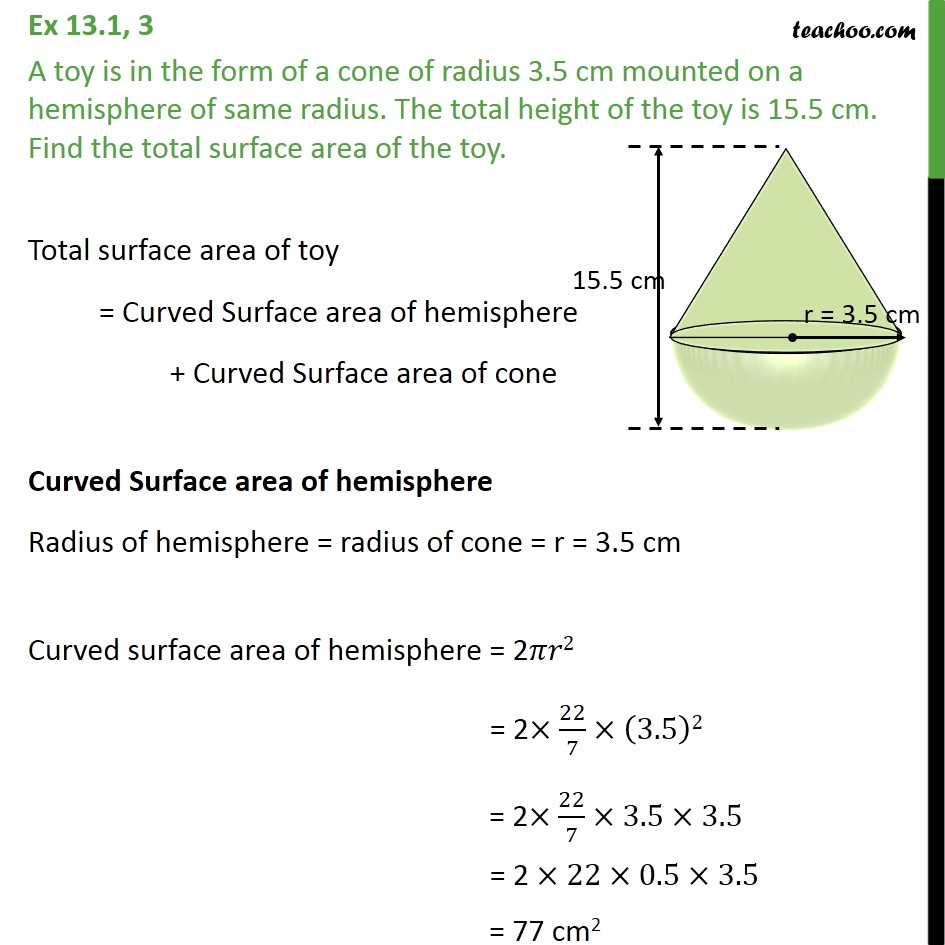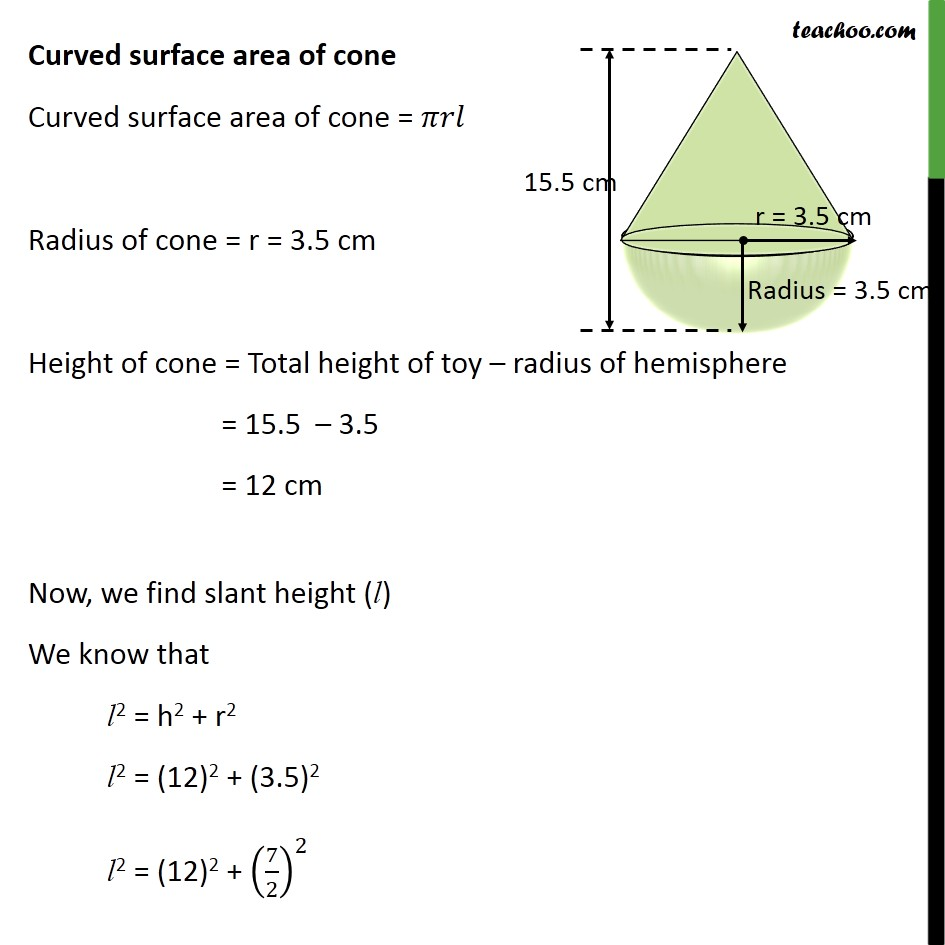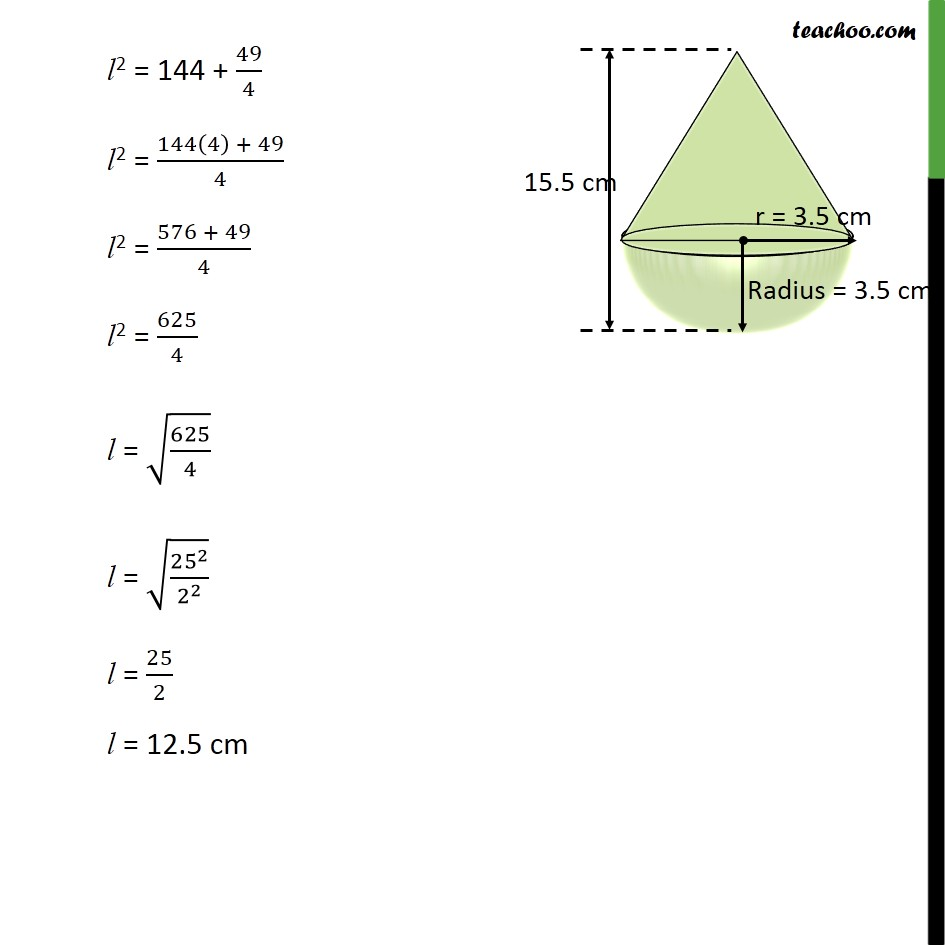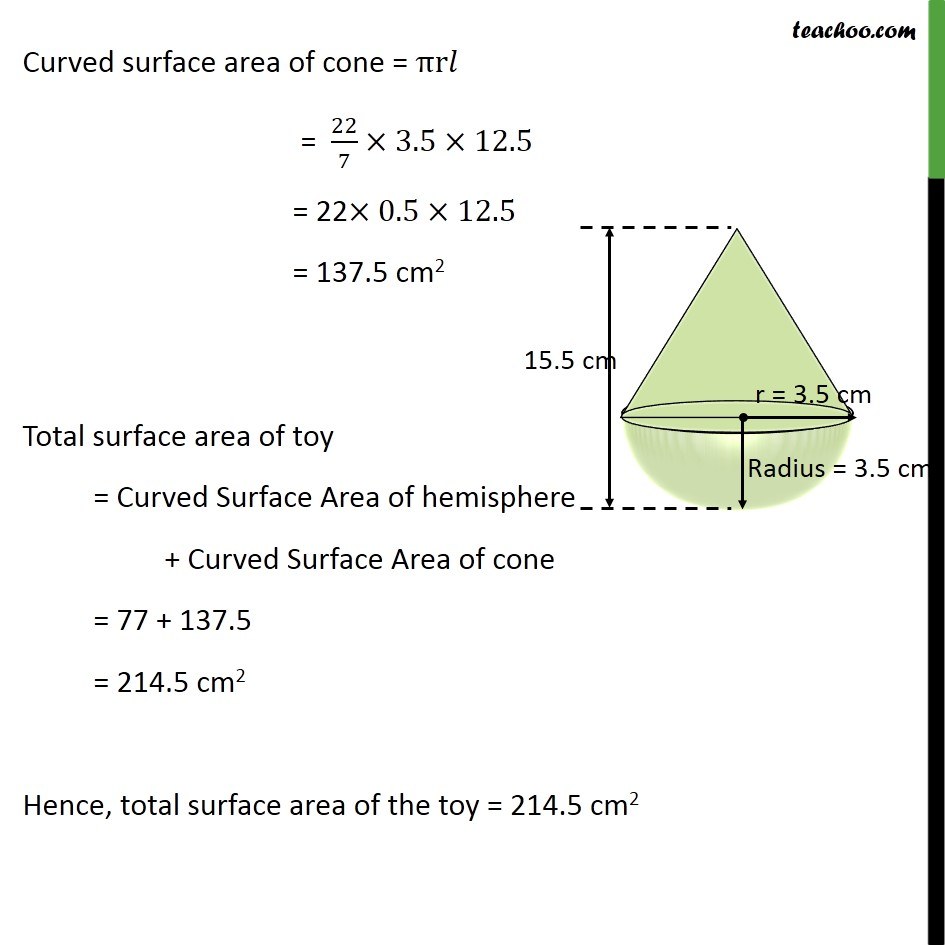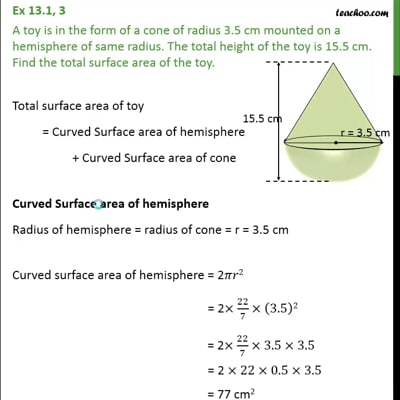This video is only available for Teachoo black users

### Transcript

Ex 13.1, 3 A toy is in the form of a cone of radius 3.5 cm mounted on a hemisphere of same radius. The total height of the toy is 15.5 cm. Find the total surface area of the toy. Total surface area of toy = Curved Surface area of hemisphere + Curved Surface area of cone Curved Surface area of hemisphere Radius of hemisphere = radius of cone = r = 3.5 cm Curved surface area of hemisphere = 2 2 = 2 22/7 (3.5)2 = 2 22/7 3.5 3.5 = 2 22 0.5 3.5 = 77 cm2 Curved surface area of cone Curved surface area of cone = Radius of cone = r = 3.5 cm Height of cone = Total height of toy radius of hemisphere = 15.5 3.5 = 12 cm Now, we find slant height (l) We know that l2 = h2 + r2 l2 = (12)2 + (3.5)2 l2 = (12)2 + (7/2)^2 l2 = 144 + 49/4 l2 = (144(4) + 49)/4 l2 = (576 + 49)/4 l2 = 625/4 l = (625/4) l = ( 25 ^2/2^2 ) l = 25/2 l = 12.5 cm Curved surface area of cone = r = 22/7 3.5 12.5 = 22 0.5 12.5 = 137.5 cm2 Total surface area of toy = Curved Surface Area of hemisphere + Curved Surface Area of cone = 77 + 137.5 = 214.5 cm2 Hence, total surface area of the toy = 214.5 cm2